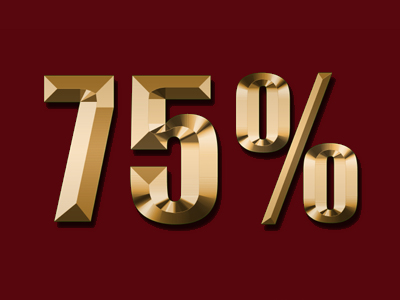75% of 200 is 150.

# Percentages 1

This Math quiz is called 'Percentages 1' and it has been written by teachers to help you if you are studying the subject at elementary school. Playing educational quizzes is an enjoyable way to learn if you are in the 3rd, 4th or 5th grade - aged 8 to 11.

It costs only \$12.50 per month to play this quiz and over 3,500 others that help you with your school work. You can subscribe on the page at Join Us

There are many ways of showing parts of whole numbers, like fractions or decimal numbers. Another way is with percentages. Percentage means the number of parts in 100. Percentage is used sometimes to give you a discount when shopping. You will come across percentages regularly in everyday life and therefore they are important to learn.

1.
What percentage is 110?
10%
20%
25%
100%
10% is also equal to 0.1
2.
Which percentage is 0.75?
25%
30%
70%
75%
0.75 and 75% are both the same as 34
3.
What is 25% equal to?
12
14
13
15
There are 4 25s in 100 so 25% is the same as 14
4.
How many percent is 0.2?
5%
10%
20%
25%
0.2 and 20% are both the same as 15
5.
Which decimal is equivalent to 25%?
0.1
0.2
0.25
0.5
0.25 and 25% are both the same as 14
6.
Which fraction is equivalent to 1%?
120
15
110
1100
Remember that percentage means the number of parts in 100 so 1% = 1100
7.
What is 50% equal to?
12
14
13
2
50% is also equal to 0.5
8.
What is 75% of 200?
75
150
225
275
75% of 100 is 75 so 75% of 200 is double 75
9.
What percentage is one whole?
25%
50%
60%
100%
Percentage means the number of parts in 100 so 100% is 100 parts - that's all of them!
10.
45% of children in a class are boys. Which percentage were girls?
45%
50%
55%
60%
The total should equal 100%
Author:  Amanda Swift